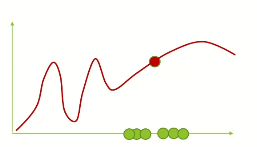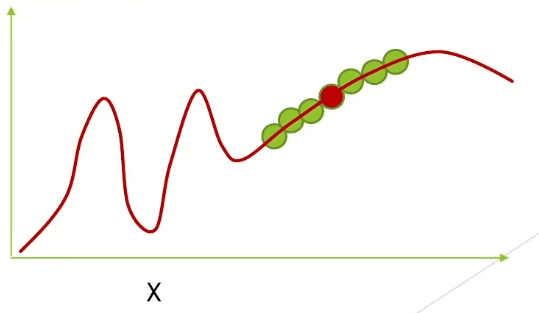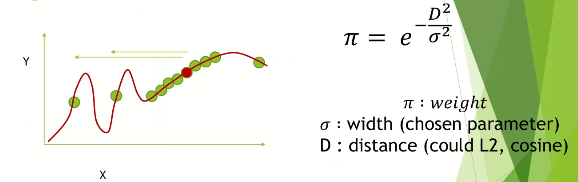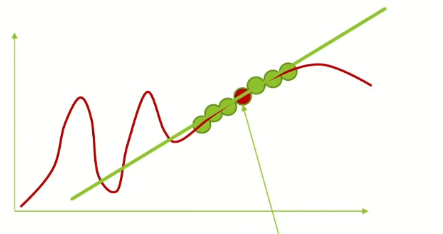Introduction

Machine learning is in the center of the latest progress in technology and is an essential tool for accurate predictions nowadays. However, most of the time we neither can clearly identify nor explain the logic behind these predictions because the model is just too complex. In those cases our machine learning model is called a ’Black Box’.

So how do we know if we can trust this model? How should we be able to trust it, when we don’t even know how it actually makes it’s predictions?

These are important questions which occur when the challenges of Model explainability are presented, especially if it is used for decision making. Users need to be confident that the model will perform well. Gaining trust in predictions through increasing transparency of a black box model, that’s one of the main goals of LIME.

So what exactly is LIME?

Ribeiro et. al (2016) puts it like this:

“LIME is an explanation technique that explains the predictions of any classifier in an interpretable and faithful manner, by learning an interpretable model locally around the prediction.”

The idea behind LIME is to approximate a complex model locally by a simple model, which has to be easy to interpret. Furthermore, we want to use that model, in our case a linear model, to explain a prediction of a particular instance, therefore it provides an understanding of the relation between the instance's components and the model's prediction.

But what does LIME actually mean?

• Local: Since the machine learning model is complex, we focus on one single prediction and find a local faithful explanation for it. Important: local fidelity does not imply global fidelity!
• Interpretable: Explanations, thus the linear models, have to be understandable for users.
• Model-agnostic: LIME treats every machine learning model as a black box. Therefore you can use LIME on any machine learning model.
• Explanations: As it says before, LIME offers an understandable explanation of one instance.

Below illustrated is the process of making an explanation for individual predictions. This specific model predicts whether a patient has the flu or not. We're applying the model to a new patient and it predicts that the patient has the flu. Can this prediction be trusted?

To verify if we can trust this model, we apply LIME. LIME returns the most important three variables in this specific prediction. The colours in our illustration indicate the evidence supporting the flu (green) and the evidence against it (red).

As just mentioned, the machine learning model predicts that this specific patient has the flu. LIME foregrounds the symptoms in the patients medical history which lead to the conclusion that this person is sick. With this deeper understanding of the model's decision-making process, a doctor is now able to verify whether he trusts the model's prediction.

But do we even need LIME?

Another important question is whether we can trust a machine learning model based on accuracy alone.

Yes, we need LIME. There is a simple reason for that: The machine learning model is a black box, therefore we literally do not know what the model is picking up on, it could be relevant, but it could also not be. Let's have a look at an example for this specific problem:

The most common question is probably: why was this prediction made or which variables caused the prediction? LIME is used as an explanation for the model, therefore it interprets how the machine learning model makes it's predictions and why a husky was classified as a wolf. The accuracy of the model is good.

However it turns out that the snow in the image was used to classify the image as 'wolf'. So if we want a snow detector, this machine learning model is the model to go for. But if we want to stick to the prior problem, it could help to add more huskies with snow in the background to your training set and more wolfs without snow in the image.

Fidelity-Interpretability Trade-Off and formal description of LIME

“for an explanation to be meaningful it must at least be locally faithful, i.e. it must correspond to how the model behaves in the vicinity of the instance being predicted”

accroding to Ribeiro et al. (2016). It is impossible for a simple model to be completely accurate unless it is the model itself and then we would not need LIME. The more complex the explanation the more accurate it is. However, we want an explanation the user can understand. So there definitely exists a Fidelity-Interpretability trade-off.

Formally, the explanation given by LIME is the following:

$$\underset{g\in G}{argmin} \zeta(x)=\mathcal{L}(f,g,\pi_{x})+\Omega(g)$$

• $$G\ \widehat{=}$$ class of potentially interpretable models such as linear models
• $$g\ \widehat{=}$$ explanation as a model
• $$\Omega(g)$$ $$\widehat{=}$$ measure of complexity (e.g. for linear models the number of non-zero weights)
• $$f:\mathbb{R}^d\rightarrow\mathbb{R}$$
f(x) is the probability (or binary indicator) that x belongs to a certain class
• $$\pi_{x}(z)$$ $$\widehat{=}$$ weight ($$\pi_{x}=e^{\frac{-D^2}{\sigma^2}}$$, where D is the distance and $$\sigma$$ the width(a chosen parameter))
• $$\mathcal{L}(f,g,\pi_{x})$$$$\widehat{=}$$ measure of how unfaithful g is in approximating f in the locality defined by $$\pi_{x}$$

For the purpose of ensuring interpretability and local accuracy, we must minimize $$\mathcal{L}(f,g,\pi_{x})$$ while having the measure of complexity low enough to be interpretable by users.

But how does LIME actually work?

We want to explain the machine learning model. Not globally, because it is too complex. We only want to explain a single prediction.

• $$X=\mathbb{R}^p$$ is the feature space
• $$X'=\mathbb{R}^{p'}$$ is the interpretable space
• $$y\in X$$ is the original representation
• $$y'\in X'$$ is the interpretable representation
• $$f: X\rightarrow\mathbb{R}$$ is the model being explained. Therefore f(x) is the probability that x belongs to a certain class.

We choose a linear model as $$g$$ in the class of potentially interpretable models.

1. Permute Data: Generate N “perturbed“ samples of the interpretable version of the instance to explain (y’). Let $$\{z'_{i} \in X'|i=1,..,N\}$$ be these observations.

2. Recover “perturbed“ data: We recover “perturbed“ observations in the original feature space X (Mapping function). Let $$\{z_{i} \in X|i=1,..,N\}$$ be the set of original representation.3. Make predictions: Make predictions on new data using the complex model.
We make a prediction of our model with the sample data in the original feature space X. Let the black box model predict the outcome of every “perturbed“ observation. Let $$\{f(z_{u})\in \mathbb{R}|i=1,...,N\}\}$$ be the set of responses.4. Weight: Compute the weight of every “perturbed“ observation, because that is how we look locally: the further away from y you are, the less weight you assign to this specific sample.
$$\rightarrow \pi_{x}=e^{-\frac{D^2}{\sigma^2}}$$, where D is the distance and $$\sigma$$ is the width (chosen parameter).

5. Select K features: Select K features best describing the black box model outcome from the perturbed dataset: $$\phi=\{(z_{i}',f(z_{i}))\in X'\times\mathbb{R}|i=1,...,N\}$$6. Linear regression: Fit a weighted linear regression model to a feature-reduced dataset composed to the K selected features.7. Interpret: Extract the coefficients from the linear model and use them as explanations for local behaviour of the black box model. A linear model is easy to interpret.

8. Check: Is the model good?: There is an easy way to see if the linear regression worked well: If $$R^2=1-\frac{\sum_{i=1}^N Y_{i}-\widehat{Y_{i}}}{\sum_{i=1}^N Y_{i}-\bar{Y}}$$ is near 1, the model is good, and bad if it is close to 0.

First Example: Text Classification

A possible representation would be a binary vector indicating the presence or absence of a word. Formally: $$X'=\{0,1\}^{p'}$$, p’ is the number of words that contains the instance being explained (the specific text). The mapping function converts a vector of 1’s or 0’s into the description used by the machine learning model, for example: We want to find an explanation for the sentence “I could move mountains“. The interpretable space would be $$X'=\{0,1\}^4$$, therefore this is one of the possible samples $$X'_{1}=(0,1,0,1)$$ and the mapping function would convert this vector to a sentence: “could mountains“.

Let's have a look at a different example: We want to classify text. We have two classes: "Christianity" and "Atheism". These classes are difficult to keep apart because they share so many words. Our machine learning model is a random forest with 500 trees. We get an accuracy of 92.4 percent and usually we would not expect the accuracy to be that high due to the prior explained reasons. If we would trust our model only based on accuracy, we would definitely trust this algorithm. However, as explained in section two, it is important to have a deeper understanding of the model and to know how it makes it's predictions.

Below is an explanation for an instance in the test set using the lime package:

This is a case where the classifier predicts the instance correctly but for the wrong reasons. This, once again, makes clear that a model should not be trusted based on accuracy alone.

Second Example: Image Classification

A possible representation would be a binary vector indicating the presence or absence of a set of contiguous similar pixels, also called super pixels.

Formally: $$X'=\{0,1\}^{p'}$$, p’ is the number of super pixels that contains the instance being explained (the specific image).

The mapping function converts a vector of 1's or 0's into the representation used by the model, in this case it colours all super pixels with the number 0 to grey, which pretends that piece of the image is missing and leaves all the super pixel with number 1 like the original.

Strengths and weaknesses of LIME

LIME gives an explanation for the prediction of tabular data, images and text, however LIME can not explain images, which can not be classified in super pixels yet. Additionally, LIME is implemented in R and Python, which makes it easy to use, but sadly LIME is not really stable yet. It "(...) showed that the explanations of two very close points varied greatly in a simulated setting.", according to Christoph Molnar in "A Guide for Making Black Box Models Explainable". That means you have to be very critical and consequently it could be difficult to fully trust LIME.

Overall, LIME can not just help answering the question "Why should I trust this model? Why made the model this specific decision?", moreover it can help choosing between competing models, detecting and improving untrustworthy models and getting insights to the model.

Show Sources